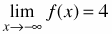### Home > CALC > Chapter 3 > Lesson 3.2.2 > Problem3-61

3-61.
1. Sketch a graph of a function that has the properties listed below. Describe anything special about this function. 3-61 HW eTool (Desmos). Homework Help ✎

•• f(1) = −1

• f(−x) = −f(x)There is a horizontal asymptote of y = 4 in the left direction.

Definition of an odd function. This means that f(−1) = 1 and there will be a horizontal asymptote of y = −4. in the right direction.

You do not have to connect this graph. (There was not a clue that said it is a continuous function.) If you choose to connect it, make sure the sketch has rotational symmetry about the origin.

Use the eTool below to create the graph.
Click the link at right for the full version of the eTool: Calc 3-61 HW eTool